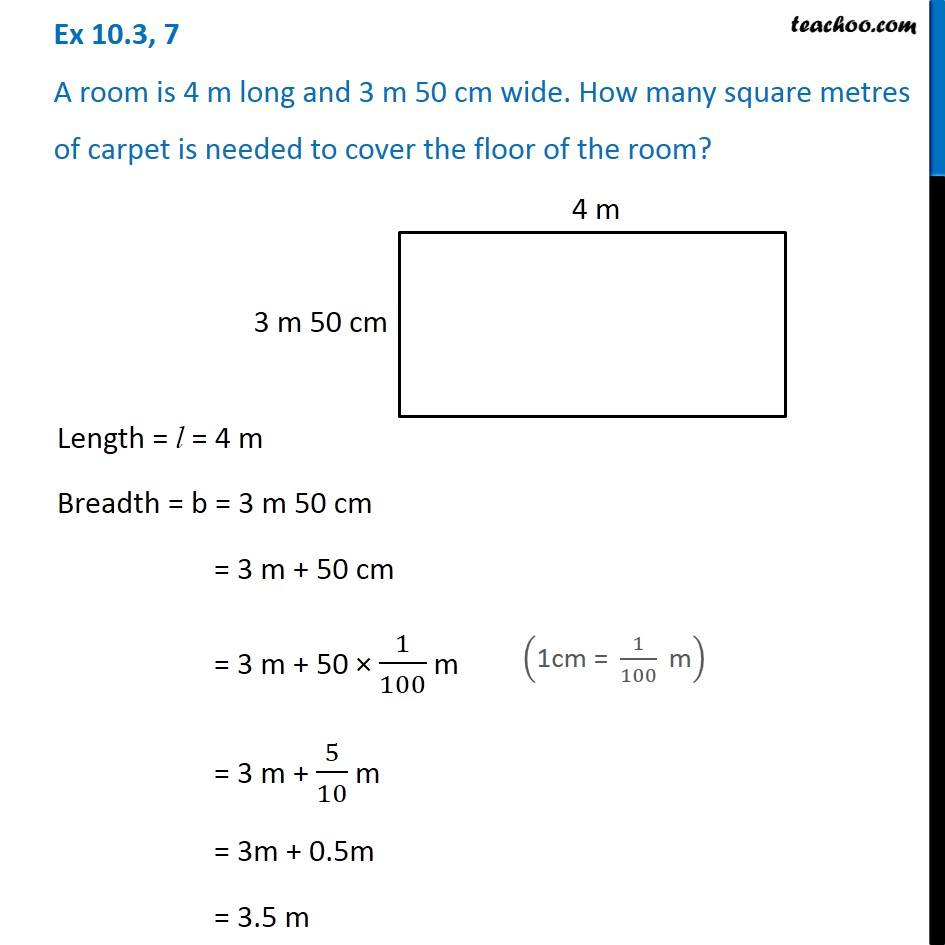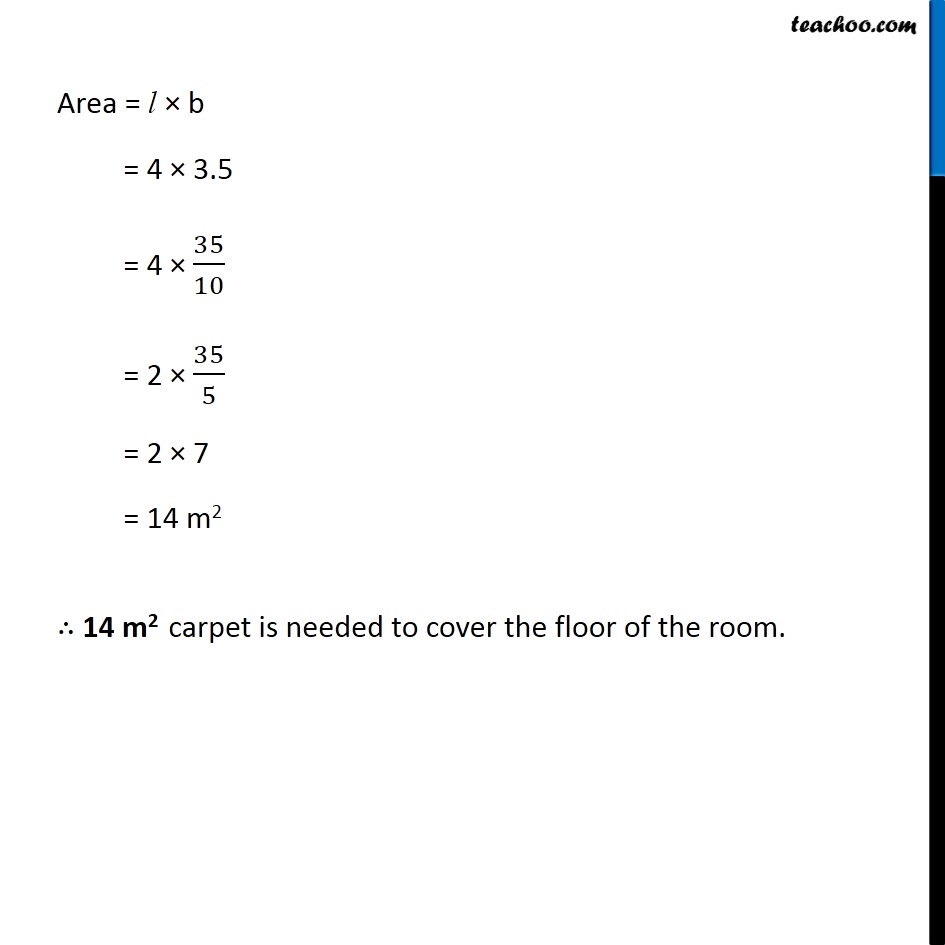Ex 10.3

Chapter 10 Class 6 Mensuration
Serial order wiseLearn in your speed, with individual attention - Teachoo Maths 1-on-1 Class

### Transcript

Ex 10.3, 7 A room is 4 m long and 3 m 50 cm wide. How many square metres of carpet is needed to cover the floor of the room? Length = l = 4 m Breadth = b = 3 m 50 cm = 3 m + 50 cm = 3 m + 50 × 1/100 m = 3 m + 5/10 m = 3m + 0.5m = 3.5 m ("1cm = " 1/100 " m" ) Area = l × b = 4 × 3.5 = 4 × 35/10 = 2 × 35/5 = 2 × 7 = 14 m2 ∴ 14 m2 carpet is needed to cover the floor of the room.